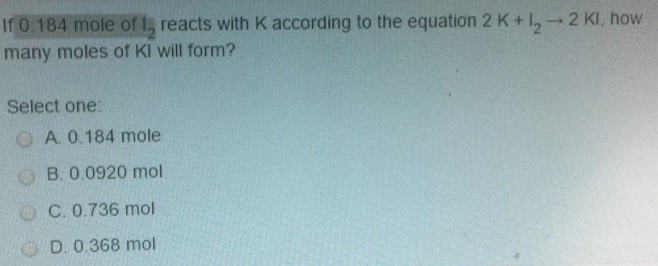# Problem: If 0.184 mole of I2 reacts with K according to the equation 2 K + I 2 → 2 KI, how many moles of KI will form? Select one:a. 0.184 moleb. 0.0920 molc. 0.736 mold. 0.368 mol

###### FREE Expert Solution
96% (276 ratings)###### Problem Details

If 0.184 mole of I2 reacts with K according to the equation 2 K + I 2 → 2 KI, how many moles of KI will form? Select one:

a. 0.184 mole

b. 0.0920 mol

c. 0.736 mol

d. 0.368 mol